### Home > PC > Chapter 9 > Lesson 9.3.3 > Problem9-134

9-134.
1.These expressions look complicated, but they are not if you know your trigonometric identities. Review the addition/subtraction formulas, double-angle formulas, and half-angle formulas in your Tool Kit. Then express each of the problems below in the form sin x or cos x. Homework Help ✎

1. sin 50°cos 20° + cos 50°sin 20°

2.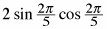3. cos110°cos50°− sin110°sin50°

4.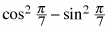5.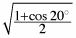6.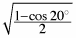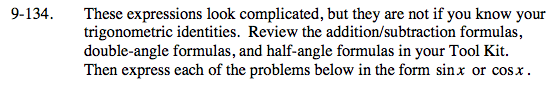Look in the index and/or glossary for these formulas.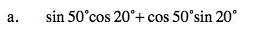Use the addition formula for sine.
sin(50° + 20°)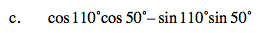Use the sum/difference formula for the cosine. Be careful of your sign.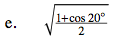Use the Half-Angle Formula for cosine.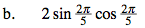Use the Double-Angle Formula for the sine.

$\text{sin}\left( 2\left( \frac{2\pi}{5} \right) \right)$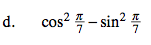Use one of the Double-Angle Formulas for the cosine.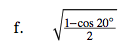Use the Half-Angle Formula for sine.